# The Development of a Modular Expansion for Raspberry Pi 3

In order to fully use your Raspberry Pi, you need to expand its functionality and make its use convenient.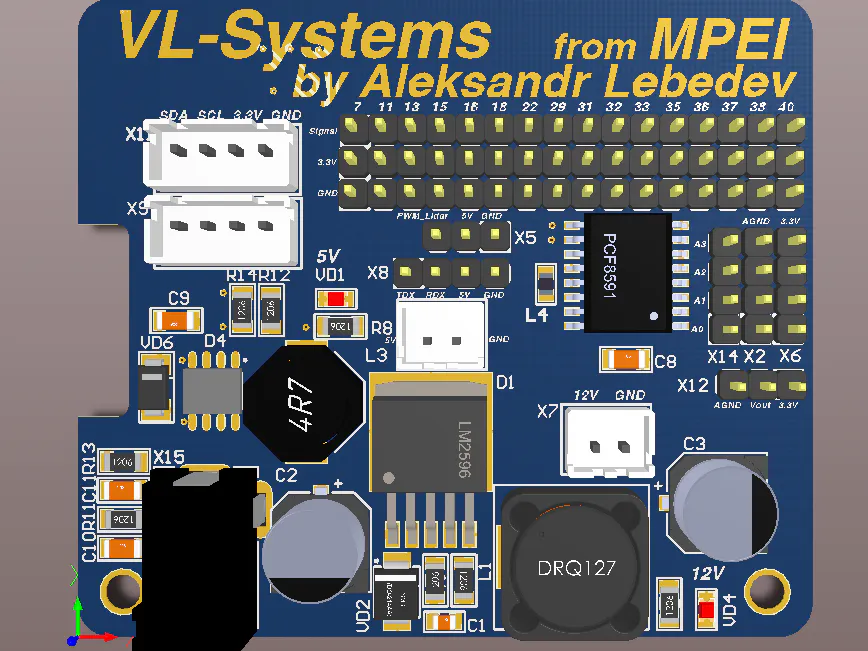## Things used in this project

### Hardware componentsPCBWay Custom PCB
×1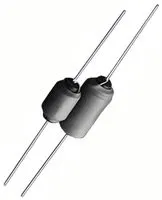Axial Leaded High Frequency Inductor, High Current
×1Capacitor 100 µF
×1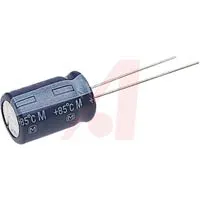Capacitor 10 µF
×1

### Software apps and online servicesCircuitMaker by Altium Circuit Maker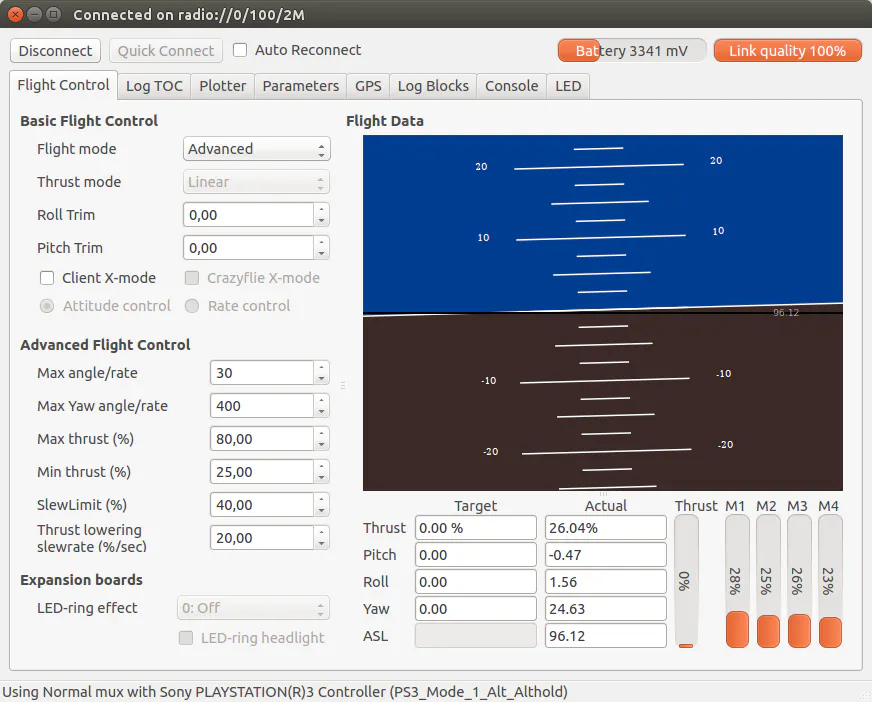Bitcraze Crazyflie Python ClientRaspberry Pi Raspbian

### Hand tools and fabrication machines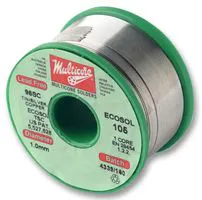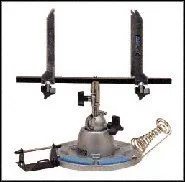Holder, PCB

## Schematics

### Schematc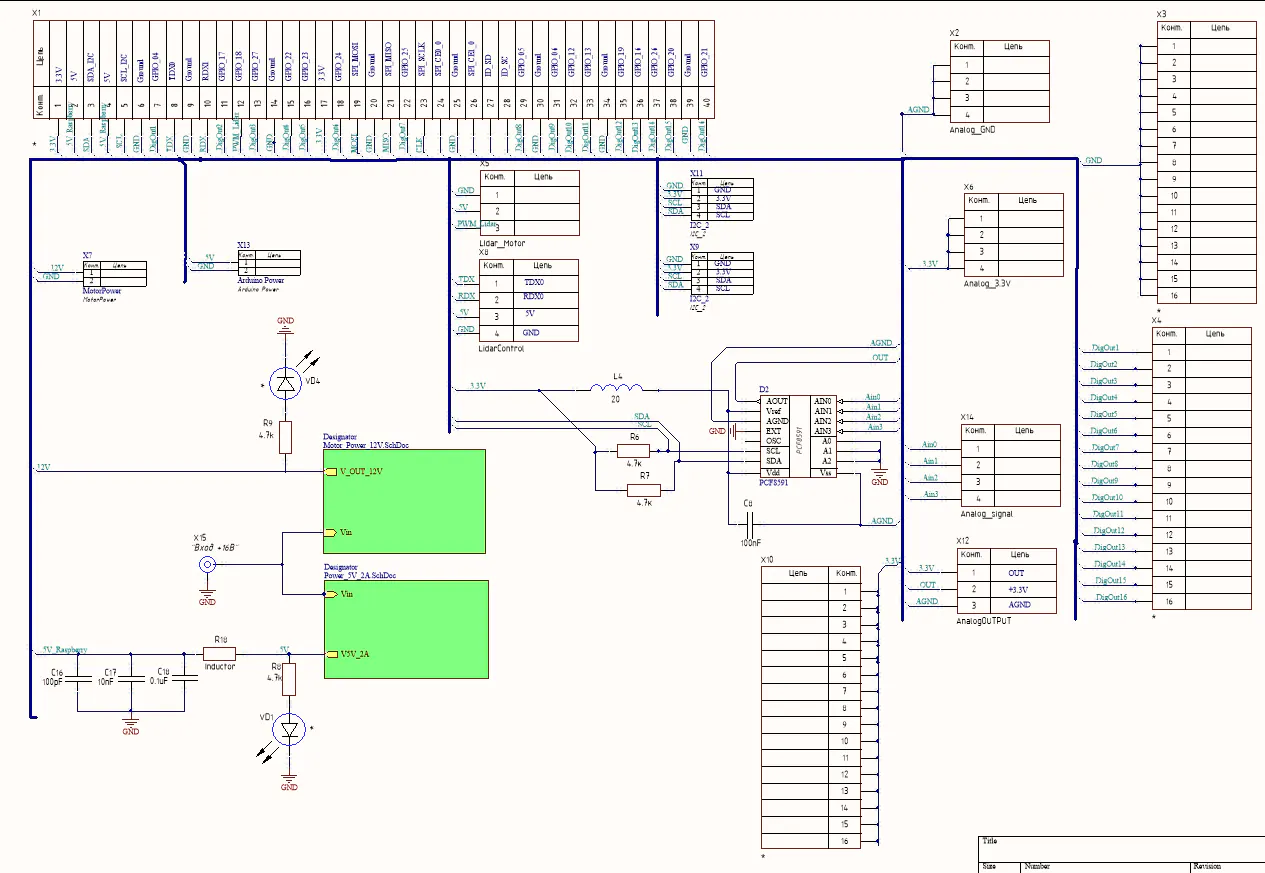## Code

### Robot.py

Python
Main porgramm for my robot
```import time
import os
import math
from array import array
import Robot_math
import Robot_CSV_Write

def frange(start,stop,step):
t=start
while t< stop:
yield t
t+=step

t=0
data=[]
for t in frange(0,8.05,0.05):
U1,U2,U3=Robot_math.Raschet(t,2)
Rotation_1,Rotation_2,Rotation_3,PWM_1,PWM_2,PWM_3=Robot_math.Evalf(U1,U2,U3)
Int_PWM_1, Float_PWM_1, Int_PWM_2, Float_PWM_2, Int_PWM_3, Float_PWM_3=Robot_math.FloatToInt(PWM_1,PWM_2,PWM_3)
Derection = array('i',[Rotation_1,Rotation_2,Rotation_3])
PWM = array('i',[Int_PWM_1,Float_PWM_1,Int_PWM_2,Float_PWM_2,Int_PWM_3,Float_PWM_3])
print(t)
print(Derection)
print(PWM)
data.append(t)
data.append(Rotation_1)
data.append(Rotation_2)
data.append(Rotation_3)
data.append(PWM_1)
data.append(PWM_2)
data.append(PWM_3)

time.sleep(0.05)
if t>=8:

Robot_CSV_Write.Write_data(data1)
Rotation_1, Rotation_2, Rotation_3, PWM_1, PWM_2, PWM_3=Robot_math.Stop()
Int_PWM_1, Float_PWM_1, Int_PWM_2, Float_PWM_2, Int_PWM_3, Float_PWM_3 = Robot_math.FloatToInt(PWM_1, PWM_2,PWM_3)
Derection = array('i', [Rotation_1, Rotation_2, Rotation_3])
PWM = array('i', [Int_PWM_1, Float_PWM_1, Int_PWM_2, Float_PWM_2, Int_PWM_3, Float_PWM_3])
print("Robot dount move")
```

## Credits

### Aleksandr

3 projects • 1 follower
I am robotics engeenier